Click to Chat

1800-1023-196

+91-120-4616500

CART 0

• 0

MY CART (5)

Use Coupon: CART20 and get 20% off on all online Study Material

ITEM
DETAILS
MRP
DISCOUNT
FINAL PRICE
Total Price: Rs.

There are no items in this cart.
Continue Shopping• Complete JEE Main/Advanced Course and Test Series
• OFFERED PRICE: Rs. 15,900
• View Details

```Chapter 6: Fractions – Exercise 6.7

Question: 1

Write each fraction. Arrange them in ascending and descending order using correct sign ‘ < ‘ , ‘ = ‘ > ‘ between the fractions: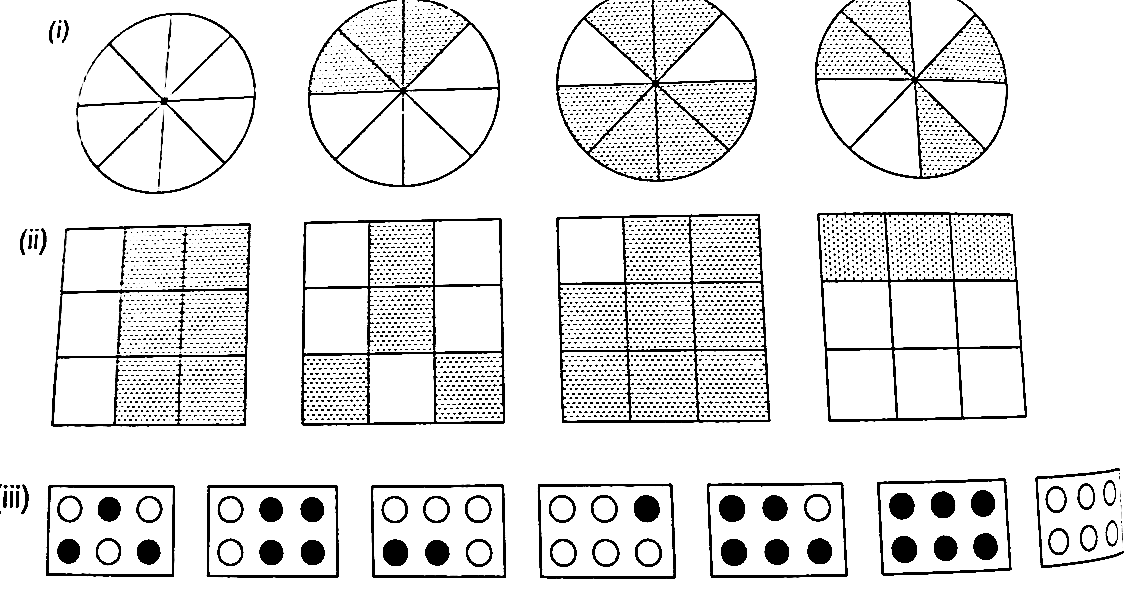Solution: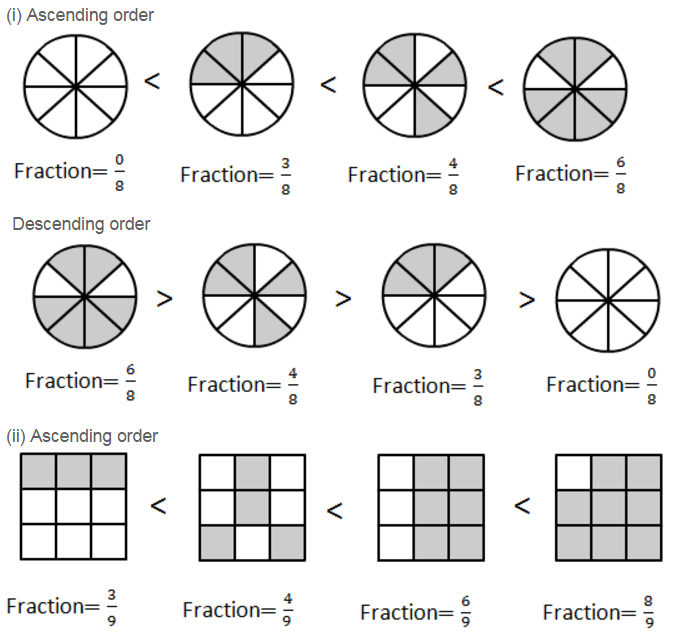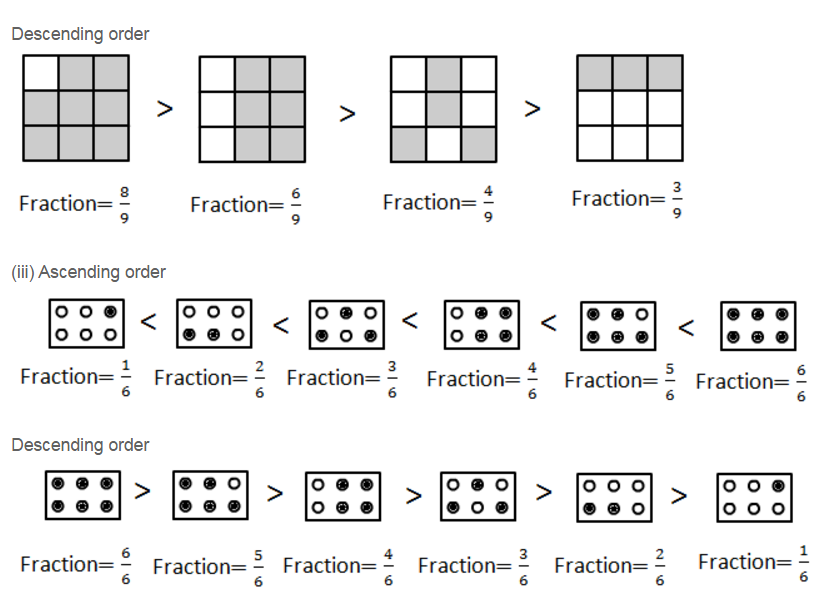Question: 2

Mark 2/6,4/6, 8/6, 6/6 on the number line and put appropriate signs between fractions given below: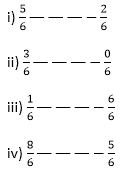Solution: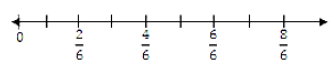i) 56 > 26 because 5 > 2 and the denominator is the same.

ii) 36 > 06 because 3 > 0 and the denominator is the same.

iii) 16 < 66 because 6 > 1 and the denominator is the same.

iv) 86 > 56 because 8 > 5 and the denominator is the same.

Question: 3

Compare the following fractions and put an appropriate: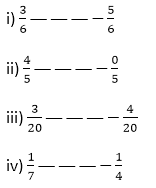Solution:

i) 36 < 56 because 3 < 5 and the denominator is the same.

ii) 45 > 05 because 4 > 0 and the denominator is the same.

iii) 320 < 420 because 3 < 4 and the denominator is the same.

iv) 17 < 14 because 7 > 4; if the numerator is the same, then the fraction that has smaller denominator is greater.

Question: 4

Compare the following fractions using the symbol > or <:

i)  6/7 and 6/11

ii) 3/7 and 5/7

iii) 2/3 and 8/12

iv) 1/5 and 4/15

v) 8/3 and 8/13

vi) 4/9 and 15/8

Solution:

i) 6/7 > 6/11 because if the numerator is the same, then the fraction with smaller denominator is greater.

ii)  3/7 < 5/7 because 3 < 5 and the denominator is the same.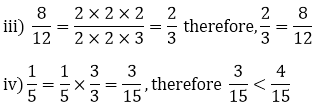(Because 3 < 4 and the denominator is the same. Therefore, 1/15 < 4/15)

v) 8/3 < 8/13 Because if the numerator is the same, then the fraction with smaller denominator is greater.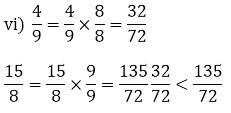(Because 135 > 32 and the denominator is the same)

Therefore, 4/9 < 15/8

Question: 5

The following fractions represent just three different numbers. Separate them in to three groups of equal fractions by changing each one to its simplest form:

i) 2/12

ii) 3/15

iii) 8/50

iv) 16/100

v) 10/60

vi) 15/75

vii) 12/60

viii) 16/96

ix) 12/75

x) 12/72

xi) 3/18

xii) 4/25

Solution:

i)  2/12

HCF of 2 & 12 is 2.

Divide both the numerator & denominator by the HCF of 2 &12ii)  3/15

HCF of 3 & 15 is 3.

Divide both the numerator & denominator by the HCF of 3 &15.iii) 8/50

HCF of 8 & 50 is 2.

Divide both the numerator & denominator by the HCF of 8 & 50.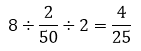iv) 16/100

HCF of 16 & 100 is 4.

Divide both the numerator & denominator by the HCF of 16 & 100.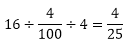v) 10/60

HCF of 10 & 60 is 10.

Divide both the numerator & denominator by the HCF of 10 & 60.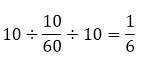vi) 15/75

HCF of 15 & 75 is 15.

Divide both the numerator & denominator by the HCF of 15 & 75.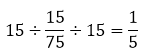vii) 12/60

HCF of 12 & 60 is 12.

Divide both the numerator & denominator by the HCF of 12 & 60.viii) 16/96

HCF of 16 & 96 is 16.

Divide both the numerator & denominator by the HCF of 16 & 96ix) 12/75

HCF of 12 & 75 is 3.

Divide both the numerator & denominator by the HCF of 12 & 75.x) 12/72

HCF of 12 & 72 is 12.

Divide both the numerator & denominator by the HCF of 12 & 72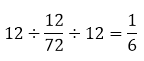xi)  3/18

HCF of 3 & 18 is 3.

Divide both the numerator & denominator by the HCF of 3 & 18.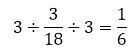xii) 4/25

HCF of 4 & 25 is 1.

Divide both the numerator & denominator by the HCF of 4 & 25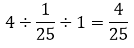Three groups of equal fractions: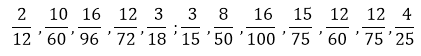Question: 6

Isha read 25 pages of a book containing 100 pages. Nagma read 1/2 of the same book. Who read less?

Solution:

Total pages in the book = 100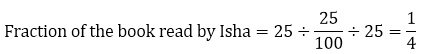(Dividing numerator & denominator by the HCF of 25 & 100)

Fraction of the book read by Nagma = 12

Now, compare 14 & 12.

LCM of 4 & 2 is 4.

Convert each fraction into equivalent fraction with 4 as its denominator.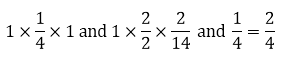Question: 7

Arrange the following fractions in the ascending order: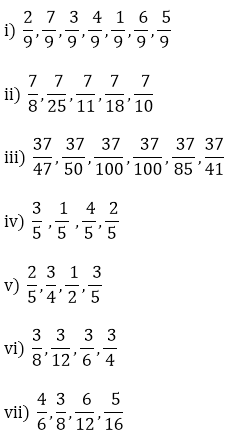Solution:

i) 2/9, 7/9, 3/9, 4/9, 1/9, 6/9, 5/9, when the denominators are the same and numerators are different, then the fraction with greater numerator has a larger value.

ii) 7/8, 7/25, 7/11, 7/18, 7/10, when numerator are the same and denominators are different, the fraction with greater denominator has a smaller value.

iii)  37/47, 37/50, 37/100, 37/100, 37/85, 37/41

When numerators are the same and denominator has a smaller value.

iv) 3/5, 1/5, 4/5, 2/5

When denominators are the same and numerators are different, then the fraction with greater numerator has a larger value.

v) LCM of 2, 4 and 5 is 20vi)  3/12, 3/8, 3/6, 3/4.

vii)  5/16, 3/8, 6/12, 4/6

Question: 8

Arrange in descending order in each of the following using symbols >:

i)  8/17, 8/9, 8/5, 8/13

ii)  5/9, 3/12, 1/3, 4/15

Solution:

i)  8/5 > 8/9 > 8/13 > 8/17

ii) 5/9 > 1/3 > 3/12 > 4/15

Question: 9

Find answers to the following. Write and indicate how you solved them.

i) Is 5/9 equal to 4/5?

ii) Is 9/16 equal to 5/9?

iii) Is 4/5 equal to 16/20?

iv) Is 1/15 equal to 4/30?

Solution:

i) No. 5 × 5 ≠ 9 × 4

ii) No. 9 × 9 ≠ 16 × 5

iii) yes. 4 × 20 = 16 × 5

iv) No. 1 × 30 = 15 × 4
```### Course Features

• 728 Video Lectures
• Revision Notes
• Previous Year Papers
• Mind Map
• Study Planner
• NCERT Solutions
• Discussion Forum
• Test paper with Video Solution